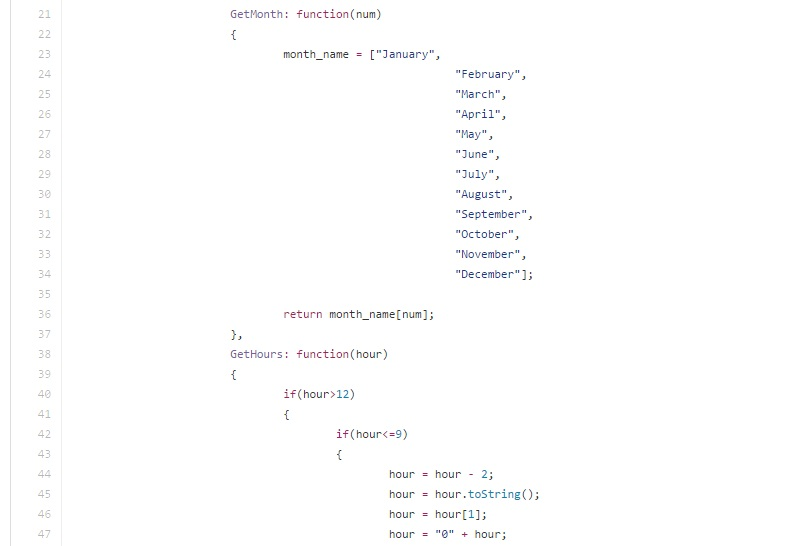## Converting javascript date to MySQL Timestamp

Home / Converting javascript date to MySQL TimestampRecently, I was assigned to a do small modification with our current project (which is written in NodeJS). The requirement needs to convert a Javascript date e.g "Sat Sep 24 2016 10:50:33 GMT-0700 (Pacific Daylight Time)" into MySQL timestamp "2016-09-24 10:53:33"

Hence, i decided to create a Javascript snippet "JSDate" to accomplish such task and also share it here.

Basically, the script accepts Javascript date object as parameter and convert it to timestamp or even timestamp parameter and convert it as readable date format.

Okay, here is the code

``````var JSDate = {

Init: function(date)
{

date = new Date(date);

month_str = this.GetMonth(date.getMonth());
month_int = this.PutZero(date.getMonth());
day = this.PutZero(date.getDate());
hour = this.GetHours(date.getHours());
minutes = this.PutZero(date.getMinutes());
seconds = this.PutZero(date.getSeconds());
ampm = this.GetAmPm(date.getHours());
year = date.getFullYear();

this.human_date = month_str+'' ''+day+'', ''+year+'' ''+hour+'':''+minutes+'':''+seconds+'' ''+ampm;
this.timestamp = year+''-''+month_int+''-''+day+'' ''+hour+'':''+minutes+'':''+seconds;

},
GetMonth: function(num)
{
month_name = ["January",
"February",
"March",
"April",
"May",
"June",
"July",
"August",
"September",
"October",
"November",
"December"];

return month_name[num];
},
GetHours: function(hour)
{
if(hour>12)
{
if(hour<=9)
{
hour = hour - 2;
hour = hour.toString();
hour = hour;
hour = "0" + hour;
}

}
return hour;
},
PutZero: function(value)
{
if(value<=9)
{
value = "0"+value;
}
return value;
},
GetAmPm: function(hour)
{
return (hour>12?''PM'':''AM'');
},
TimeStamp()
{
return this.timestamp;
},
HumanDate()
{
return this.human_date;
}}``````

# Basic Usage

JSDate.Init(new Date());

JSDate.TimeStamp(); // Output: Current Date in timestamp

# MySQL timestamp as parameter

JSDate.Init("2015-01-01 11:00:00");

// Return Timestamp Date
JSDate.TimeStamp(); // Output: 2015-01-01 11:00:00